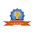# vs.eyeandcontacts.com

## Chapter 8 Conditional Control Statements

Brain Developers
A. Fill in the blanks:
1. A ______________ has a unique name, a type, and a size, which is used to identify it in a program.
2. A string variable contains values, _____________ or ______________ within double quotes.
3. IF-THEN statement is used for making _______________ as well as _________________.
4. If the conditional specified after IF is true, then the instructor after ____________ is executed.
5. _____________________ is a conditional decision making statement.
6. _______________ statements help to control the flow of a program.

1. A variable has a unique name, a type, and a size, which is used to identify it in a program.
2. A string variable contains values, symbol or text within double quotes.
3. IF-THEN statement is used for making decision as well as comparison.
4. If the conditional specified after IF is true, then the instructor after Then is executed.
5. IF THEN ELSE is a conditional decision making statement.
6. Control statements help to control the flow of a program.

B. State True or False:
1. Constants can change their values during execution of a program. (False)
Correct Answer: Constants cannot change their values during execution of a program./Variables can change their values during execution of a program.
2. Numeric variables can only hold numbers. (True)
3. The condition IF THEN ELSE statements is given by the logical operators. (False)
Correct Answer: The condition IF THEN ELSE statements is not given by the logical operators.
4. GOTO statement transfers the program control from one statement to another. (True)
5. In READ-DATA statement, the data is provided in a program at the time of execution. (False)
Correct Answer: In INPUT statement, the data is provided in a program at the time of execution.

Application Based Questions:
1. Vishal has created a program in QB64. He wants that the output of the program should be displayed subjected to true and false condition. Suggest him the appropriate statement.
The appropriate statement for Vishal will be IF…THEN statement.

2. Smriti wants to give output of a program in infinite loop. Which statement will you suggest her to use?
She can use GOTO statement for giving output of a program in infinite loop.

D. Multiple Choice Questions:
1. ___________ sign in used to represent an alphabet in a string.
a) +
b) \$
c) ?
b) \$

2. ________ statement is used to making decision based on comparisons.
a) PRINT
b) INPUT
c) IF…THEN
c) IF…THEN

3. In IF…THEN…ELSE statement, if the condition is False, then the ___________ statement will be processed.
a) ELSE
b) IF
c) THEN
a) ELSE

4. _____________ statement is used to transfer the program control from one statement to another.
a) PRINT
b) IF THEN
c) GOTO
c) GOTO

5. _____________ statement is used when we want to have more choices in the IF…THEN statement.
a) ELSEIF
b) GOTO
c) IF…THEN…ELSE
a) ELSEIF

E. Identify the errors:
1. IF A=5 Rs THEN GOTO START
IF A=S THEN GO TO START or IF A\$= “5 Rs” THEN GO TO START

2. IF A>B THEN PRINT A IS GREATER
IF A>B THEN PRINT “A IS GREATER”

3. IF A=10A THEN PRINT A\$ ELSE PRINT B
IF A=10 THEN PRINT A ELSE PRINT B

4. IF Y\$=“KABIR” THEN GOTO A: OTHERWISE GOTO B:
IF Y\$=“KABIR” THEN GO TO A ELSE TO B

5. IF X=“SUNDAY” THEN PRINT, “HAVE FUN” ELSE PRINT, “FOLLOW THE ROUTINE”
IF X\$=“SUNDAY” THEN PRINT “HAVE FUN” ELSE PRINT “FOLLOW THE ROUTINE”

1. What do you understand by constants and variables?

• Constants are the values that do not change during the execution of a program. Constants are of two types: Numeric Constants and Character Constants.
• A variable is a named location in the memory of computer that stores data temporarily. It can hold one value at a time and can accept different values during the execution of the program. Variables are of two types: Numeric and String.

2. Describe the use of Control statements.
The statements that help to control the flow of a program and change the order of execution of statements based upon a given condition are known as Control Statements. There are various control statements in QB64, like IF...THEN, GOTO, IF...THEN...ELSE, etc.

3. Define IF...THEN statement with the help of an example.
The IF-THEN statement is the most basic type of control statement. It tells the program to execute a set of statements only if the condition evaluates to true. Example: IFX > Y THEN C = C+1 It means that if X is greater than Y, then 1 is added to the value of C.

4. Briefly explain the conditional statement IF-THEN-ELSE.
IF-THEN-ELSE is a two-way decision making statement. If the condition given after IF part is true, statement(s} specified after THEN gets executed. But if the condition is False, the statements after ELSE part will be processed.
Syntax: IF THEN ELSE

5. Explain the use of END IF statement with the help of an example.
END IF statement is required after the use of multiple line IF, ELSE IF or ELSE statement blocks. It terminates the IF...THEN....ELSE block. It should be written before the END statement.
Example: CLS
INPUT X
IF X > 1 THEN
PRINT "X Is Greater Than 10"
ELSE
PRINT "X Is Not Greater Than 10"
END IF

6. What is the use of GOTO statement? Explain with an example.
A GOTO statement is referred to as unconditional transfer control. GOTO statement is used to transfer the program control from one statement to another in a program.
Syntax: GOTO
Example: GOTO Start:

1.The answers are very helpful for my computer homework. Thankyou very much ! :)

1.Welcome! If you found our website helpful do share it with your friends! You can also follow us on:
• Pinterest: https://in.pinterest.com/EducationWithVS

2.1.Thanks! Do share and subscribe our site for latest updates!

3.very helpful to me and very good to understand

1.